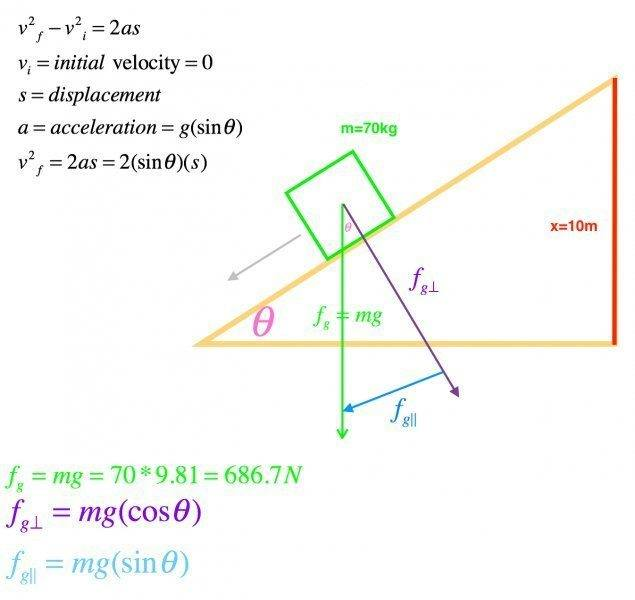# Velocity down an incline

keithcuda

## Homework Statement

A 75KG object, goes down a slide that is 10m tall. What is the speed of the object at the halfway mark and the speed at the bottom of the slide. The object starts at rest and there is no friction.

## Homework Equations

v2f - v2i = 2as

3. The Attempt at a Solution [/B]
attached pic of where I am at on the problemHomework Helper
You have the equation: v2f - v2i = 2as. Note that s is the displacement along the slope now. How long is it in terms of the angle if the height of the slope is 10 m?

keithcuda
You have the equation: v2f - v2i = 2as. Note that s is the displacement along the slope now. How long is it in terms of the angle if the height of the slope is 10 m?
sinθ=(10/h)
h equaling the hypotenuse = the slope

Homework Helper
No, h=10 m, and s is the hypotenuse. Look at the picture.

keithcuda
No, h=10 m, and s is the hypotenuse. Look at the picture.
ok so,

sin(θ)=(10/s)

Homework Helper
Isolate s and use it in the equation v2f - v2i = 2as.

keithcuda
so to isolate s, do you mean something like this?

s=10sin(θ)

Homework Helper
so to isolate s, do you mean something like this?

s=10sin(θ)
Something like that, but not quite. Is it more clear if I say solve the equation sin(θ)=(10/s) for s?

keithcuda
Something like that, but not quite. Is it more clear if I say solve the equation sin(θ)=(10/s) for s?
more like this?
s=10/sin(θ)

I'm sorry, this stuff has me so confused... These online classes aren't really meeting my expectations. :(

Last edited:
Homework Helper
Yes.
Determine the acceleration a now.

keithcuda
Yes.
Determine the acceleration a now.
well, acceleration = gsin(θ)
so, (9.81)sin(θ)=a

Homework Helper
Use a and s in the formula v2f - v2i = 2as.

keithcuda
well v2i = 0 since the object starts at rest
v2f = 2((9.81)sin(θ)) (10/sin(θ))

Homework Helper
Good! Evaluate.

keithcuda
I'm not sure if this is right, but it's a shot in the dark...

v2f =2 ((9.81)sin(θ) (10/sin(θ))
umm??? sin(θ) cancels out on each side leaving, 2(9.81)(10) = 196.2
v2f = 196.2
vf = √196.2 = 14m/s

is this even close?

Homework Helper
It is correct. That is the speed at the bottom of the slope when the whole length is covered. What is the speed, when the object is halfway down the slope?

keithcuda
It is correct. That is the speed at the bottom of the slope when the whole length is covered. What is the speed, when the object is halfway down the slope?
lol i would say half of that? Thats just me guessing...

Homework Helper
lol i would say half of that? Thats just me guessing...
NO!!!!!!!!!!!!! what is the new distance s(half) if it is half the previous one?

keithcuda
NO!!!!!!!!!!!!! what is the new distance s(half) if it is half the previous one?
haha I knew it wouldn't be that easy ;)

v2f = 2 * 9.81(10 * .5)
vf = √98.1
vf = 9.9m/s

Homework Helper
You did it!

•keithcuda
keithcuda
I feel the need to celebrate!!!
Thank you very much!

When I started this class, I really thought it would be one of my favorite subjects. I like it, but it really has me scratching my head.

I greatly appreciate it!
Merry Christmas!!

Cheers!

Homework Helper
Merry Christmas to you, too. :)